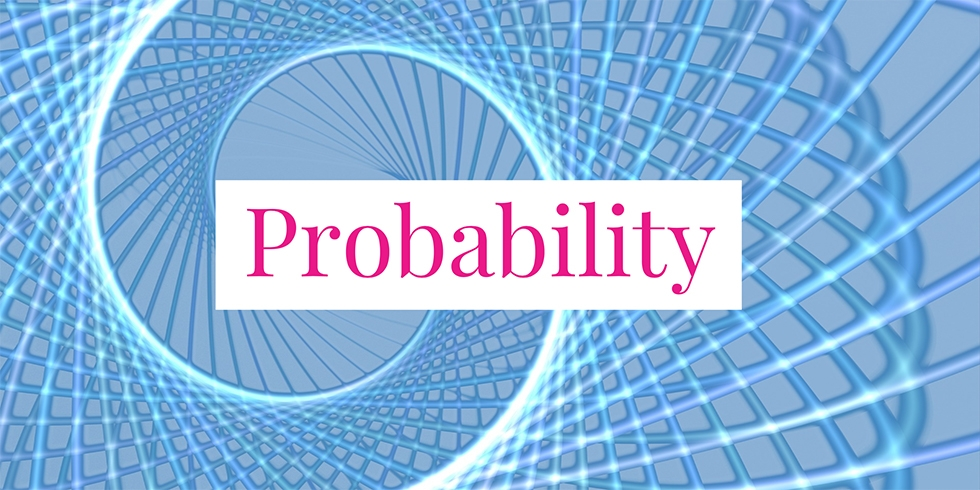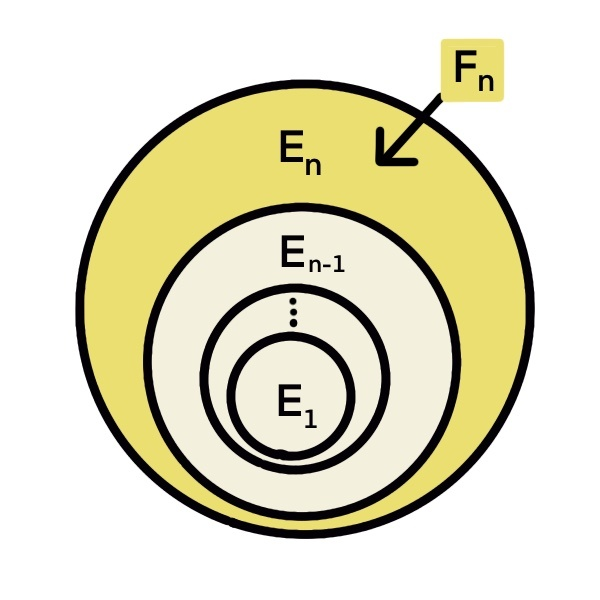# Interchangeability of Limits and Probability of Increasing or Decreasing Sequence of Events## Problem 744

A sequence of events $\{E_n\}_{n \geq 1}$ is said to be increasing if it satisfies the ascending condition
$E_1 \subset E_2 \subset \cdots \subset E_n \subset \cdots.$ Also, a sequence $\{E_n\}_{n \geq 1}$ is called decreasing if it satisfies the descending condition
$E_1 \supset E_2 \supset \cdots \supset E_n \supset \cdots.$

When $\{E_n\}_{n \geq 1}$ is an increasing sequence, we define a new event denoted by $\lim_{n \to \infty} E_n$ by
$\lim_{n \to \infty} E_n := \bigcup_{n=1}^{\infty} E_n.$

Also, when $\{E_n\}_{n \geq 1}$ is a decreasing sequence, we define a new event denoted by $\lim_{n \to \infty} E_n$ by
$\lim_{n \to \infty} E_n := \bigcap_{n=1}^{\infty} E_n.$

(1) Suppose that $\{E_n\}_{n \geq 1}$ is an increasing sequence of events. Then prove the equality of probabilities
$\lim_{n \to \infty} P(E_n) = P\left(\lim_{n \to \infty} E_n \right).$ Hence, the limit and the probability are interchangeable.

(2) Suppose that $\{E_n\}_{n \geq 1}$ is a decreasing sequence of events. Then prove the equality of probabilities
$\lim_{n \to \infty} P(E_n) = P\left(\lim_{n \to \infty} E_n \right).$Add to solve later

## Proof.

### Proof of (1)

Let $\{E_n\}_{n \geq 1}$ be an increasing sequence of events. Then we define new events $F_n$ as follows.
\begin{align*}
F_1 &= E_1\\
F_n & = E_n E_{n-1}^c && \text{ for any } n \geq 2
\end{align*}
Here, $E_{n-1}^c$ denotes the complement of $E_{n-1}$.
The event $F_n$ is depicted as a yellow region in the figure below.From the figure, we can see that the events $\{F_n\}_{n \geq 1}$ are mutually exclusive, that is, $F_iF_j = \emptyset$ for any $i \neq j$.
Furthermore, note that
$\bigcup_{i=1}^n F_i = \bigcup_{i=1}^n E_i \tag{*}$ for any $n$, and
$\bigcup_{i=1}^{\infty} F_i = \bigcup_{i=1}^{\infty} E_i. \tag{**}$ \end{enumerate}

Now we proceed to the main part and prove the equality
$\lim_{n \to \infty} P(E_n) = P \left(\lim_{n \to \infty} E_n \right).$ We start with the right-hand-side and show that it is equal to the left-hand-side.
\begin{align*}
P\left(\lim_{n \to \infty} E_i \right) &= P \left(\bigcup_{i=1}^{\infty} E_n \right) && \text{ definition of } \lim_{n \to \infty} E_n\6pt] &= P \left(\bigcup_{i=1}^{\infty} F_i \right) && \text{ by } (**) \\[6pt] &= \sum_{i=1}^{\infty} P(F_i) && \text{ by one of the axioms of probability} \\ & && \text{ with mutually exclusive events } \{F_n\}\\[6pt] &= \lim_{n \to \infty} \sum_{i=1}^n P(F_i) \\[6pt] &= \lim_{n \to \infty} P\left( \bigcup_{i=1}^n F_i \right) && \text{ by additivity with mutually exclusive events } \{F_n\}\\[6pt] &= \lim_{n \to \infty} P\left(\bigcup_{i=1}^n E_i \right) && \text{ by } (*)\\[6pt] &= \lim_{n \to \infty} P(E_n) && \text{as \{E_n\} is increasing}. \end{align*} This proves the desired equality. ### Proof of (2) Now, we consider a decreasing sequence of events \{E_n\}_{n\geq 1}. As \{E_n\}_{n\geq 1} is decreasing, its complement \{E_n^c\}_{n \geq 1} is increasing. Thus, by the result of part (1), we see that \[\lim_{n \to \infty} P(E_n^c) = P \left(\lim_{n \to \infty} E_n^c \right). \tag{***} By definition, we have
$\lim_{n \to \infty} E_n^c = \bigcup_{n=1}^{\infty} E_n^c = \left(\bigcap_{n=1}^{\infty} E_n \right)^c.$

It follows that the right-hand-side of the equality (***) becomes
\begin{align*}
P \left(\lim_{n \to \infty} E_n^c \right) &= P\left(\left(\bigcap_{n=1}^{\infty} E_n \right)^c \right)\6pt] &= 1 – P\left(\bigcap_{n=1}^{\infty} E_n \right)\\[6pt] &= 1 – P\left(\lim_{n \to \infty} E_n \right). \end{align*} On the other hand, the left-hand-side of the equality (***) becomes \begin{align*} \lim_{n \to \infty} P(E_n^c) &= \lim_{n \to \infty} \left( 1 – P(E_n) \right)\\[6pt] &= 1 – \lim_{n \to \infty} P(E_n). \end{align*} Combining these results yields \[1 – \lim_{n \to \infty} P(E_n) = 1 – P\left(\lim_{n \to \infty} E_n \right). Equivalently, we have
$\lim_{n \to \infty} P(F_n) = P\left(\lim_{n \to \infty} E_n \right),$ which is the desired equality. This completes the proof.Add to solve later

### More from my site

#### You may also like...

This site uses Akismet to reduce spam. Learn how your comment data is processed.

###### More in Probability##### Linearity of Expectations E(X+Y) = E(X) + E(Y)

Let $X, Y$ be discrete random variables. Prove the linearity of expectations described as $E(X+Y) = E(X) + E(Y).$

Close